# Mathematics Important Questions for Class 12 Board 2019

Here are some good maths important questions for Class 12 Board 2019.

Question 1)

The x-coordinate of a point on the line joining the points P(2, 2, 1) and Q(5, 1, – 2) is 4. Find its z-coordinate

Question 2)

Write the principle value of  tan-1(1) +cos-1(-1/2)

Question 3)
A fair coin is tossed 8 times, find the probability of

Question 4)
Three cards are drawn successively with replacement from a well shuffled pack of
52 cards. Find the mean and variance of the number of red cards

Question 5)

There are three coins. One coin is two headed coin.Second coin is biased one which comes up tail 25% times and third coin is unbiased one.One of the three coin is chosen at random and tossed and head comes. what is the probability that it was the two-headed coin?

Question 6)

Determine the values of ‘a’ and ‘b’ such that the following function is continuous
at x = 0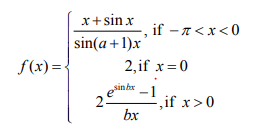Question 7)

Determine the value of ‘k’ for which the following function is continuous
at x = 3 :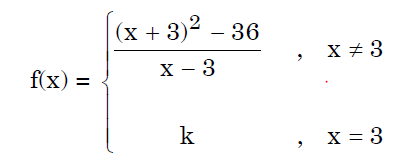Question 8)

Find the value of k for which the function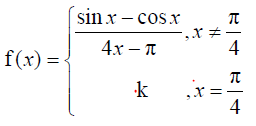is continuous at x=?/4

Question 9)

if xy + yx= ab find dy/dx

Question 10)

Find the intervals in which the function f given by
f(x) = sin x + cos x, 0 ? x ? 2?
is strictly increasing or strictly decreasing.

Question 11)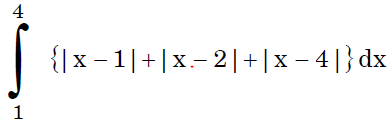Question 12)

Find the intervals in which the function given by

f(x) = 2x3 – 3x2 – 36x + 7 is

(a) Strictly increasing

(b) Strictly decreasing

Question 13)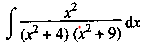Question 14)

Find the value of the below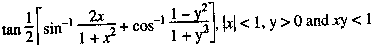Question 15)

If a ,b and c are mutually perpendicular vectors of equal magnitudes, find the angles which the vector 2a +b+ 2c makes with the vectors a ,b and c .

Question 16)
Solve the following linear programming problem graphically:
Minimize: z = 3x + 9y
When: x + 3y ? 60
x + y ? 10
x?   y
x ? 0, y ? 0

Question 17)

There are 4 cards numbered 1, 3, 5 and 7, one number on one card. Two cards are drawn at random without replacement. Let X denote the sum of the numbers on the two drawn cards. Find the mean and variance of X

Question 18)

Using Integration, find the area of the following region

{(x, y) : y2 ? ax, x2 + y2 ? 2ax,a > 0}

Question 19)

Find the equation of the plane passing through the point (–1, 3, 2) and perpendicular to
each of the planes x + 2y + 3z = 5 and 3x + 3y + z = 0

Question 20)

Let f : R – (-4/3) -> R be function defined as f(x)=4x/3x + 4. Show that f is a one-one
function. Also check whether f is an onto function or not. Hence find f-1 in
(Range of f) -> R – (-4/3)

Question 21)

$\int { \frac {x^2 -1}{x^4 +1}} dx$

Question 22)

$\int {\left (\sqrt {tanx} + \sqrt {cot x} \right)} dx$